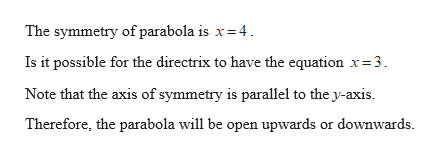# If the axis of symmetry of a parabola is given by x = 4, is it possible for the directrix to have the equation x = 3? Explain.

Question
4 views

If the axis of symmetry of a parabola is given by x = 4, is it possible for the directrix to have the equation x = 3? Explain.

check_circle

Step 1help_outlineImage TranscriptioncloseThe symmetry of parabola is x= 4. Is it possible for the directrix to have the equation x=3. Note that the axis of symmetry is parallel to the y-axis. Therefore, the parabola will be open upwards or downwards. fullscreen

### Want to see the full answer?

See Solution

#### Want to see this answer and more?

Solutions are written by subject experts who are available 24/7. Questions are typically answered within 1 hour.*

See Solution
*Response times may vary by subject and question.
Tagged in
MathAlgebra

### Other